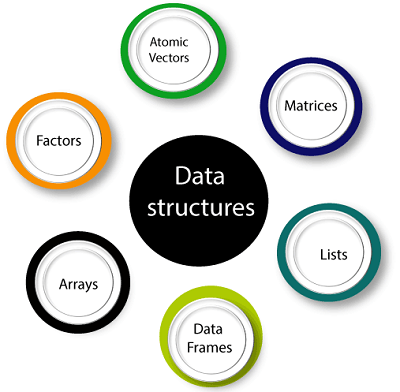# Data Structures in R Programming

Data structures are very important to understand. Data structure are the objects which we will manipulate in our day-to-day basis in R. Dealing with object conversions is the most common sources of despairs for beginners. We can say that everything in R is an object.

R has many data structures, which include:1. Atomic vector
2. List
3. Array
4. Matrices
5. Data Frame
6. Factors

### Vectors

A vector is the basic data structure in R, or we can say vectors are the most basic R data objects. There are six types of atomic vectors such as logical, integer, character, double, and raw. “A vector is a collection of elements which is most commonly of mode character, integer, logical or numeric” A vector can be one of the following two types:

1. Atomic vector
2. Lists

### List

In R, the list is the container. Unlike an atomic vector, the list is not restricted to be a single mode. A list contains a mixture of data types. The list is also known as generic vectors because the element of the list can be of any type of R object. "A list is a special type of vector in which each element can be a different type."

We can create a list with the help of list() or as.list(). We can use vector() to create a required length empty list.

### Arrays

There is another type of data objects which can store data in more than two dimensions known as arrays. “An array is a collection of a similar data type with contiguous memory allocation.” Suppose, if we create an array of dimension (2, 3, 4) then it creates four rectangular matrices of two rows and three columns.

In R, an array is created with the help of array() function. This function takes a vector as an input and uses the value in the dim parameter to create an array.

### Matrices

A matrix is an R object in which the elements are arranged in a two-dimensional rectangular layout. In the matrix, elements of the same atomic types are contained. For mathematical calculation, this can use a matrix containing the numeric element. A matrix is created with the help of the matrix() function in R.

Syntax

The basic syntax of creating a matrix is as follows:

1. matrix(data, no_row, no_col, by_row, dim_name)

### Data Frames

A data frame is a two-dimensional array-like structure, or we can say it is a table in which each column contains the value of one variable, and row contains the set of value from each column.

There are the following characteristics of a data frame:

1. The column name will be non-empty.
2. The row names will be unique.
3. A data frame stored numeric, factor or character type data.
4. Each column will contain same number of data items.

### Factors

Factors are also data objects that are used to categorize the data and store it as levels. Factors can store both strings and integers. Columns have a limited number of unique values so that factors are very useful in columns. It is very useful in data analysis for statistical modeling.

Factors are created with the help of factor() function by taking a vector as an input parameter.